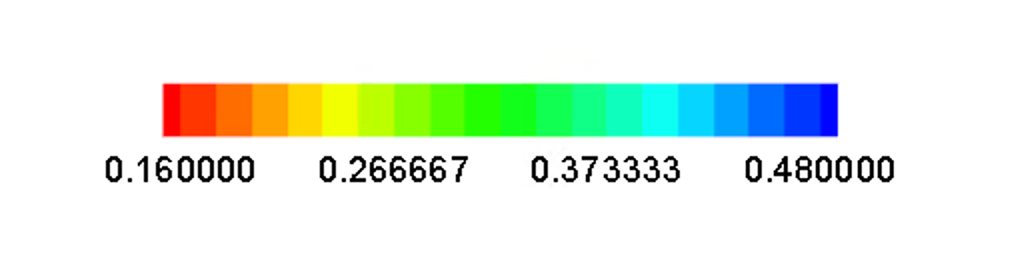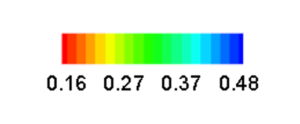# Adjusting the Number of Decimal Places in Tecplot RS Contour Legends

Problem:

In Tecplot RS, my contour legend displays too many digits (Figure 1). How do I decrease the number of digits shown in the legend?Figure 1

Solution:

Using the macro below, you can change the contour label precision of the contour plot. In order to run this .mcr file you will need to:
1. Copy the macro text below and paste it into a text editor.
2. Save this file as a .mcr file (Tecplot macro file).
3. Open up Tecplot RS, go to Macro>Play Macro…, and navigate to wherever you saved the .mcr file above.
See the result after running the macro file in Figure 2. In the example below, we set the precision to 2, but you can change the precision as needed.
```#!MC 1410
\$!GlobalContour 1  Labels
{
NumFormat
{
Formatting = FixedFloat
Precision = 2
}
}```Figure 2